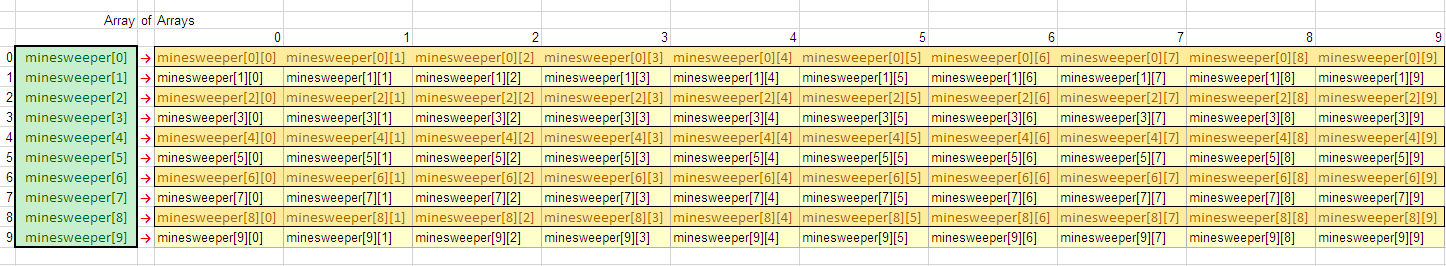jQuery and Javascript Tutorials and Code Examples Development resources, articles, tutorials, code samples and tools and downloads for ASP.Net, SQL Server, R Script, Windows, Windows Phone, AWS, SAP HANA and ABAP, like SAP UI5, Screen Personas, etc.
 Home Articles News IT Jobs Tools Sample Chapters Trainers Blogs Forums Photos Files

# Javascript MultiDimensional Array in Javascript Array example codes

Javascript MultiDimensional array in Javascript development is very common in web applications. For example web developer wants to create Javascript game with a board 10x20, let's say Javascript minesweeper game. Then easiest way to simulate the real world game board is to create Javascript multi-dimensional array and store game data on this Javascript array.

Although there is not a build-in multi dimension array definition in Javascript, web developers can use a simple trick to define arrays with multiple dimension. The trick is to create an array with the length of the first dimension. Then within a Javascript For Loop iterating each item in first array, developer can set current item as a new array with a length of second dimension. This method will enable two-dimensional Javascript array definition in web programming. To summarize, multidimensional arrays are Javascript array of arrays created by using Javascript For Loop and simple arrays in Javascript.

Here is our first Javascript code example which can be used to define multi dimensional Javascript array. Our first Javascript tutorial example will create two dimensional array (10,20) for a minesweeper board.

``` var m = 10; var n = 20; var minesweeper = new Array(m); for (i = 0; i < m; i = i + 1) {  minesweeper[i] = new Array(n); } ```

If Javascript programmer wants to place game figures, the bombs in minesweeper board multi-dimensional array, following js code can be used. Assume that the array value 'X' identifies a bomb on the board. Or to make it nicer like the Windows 7 version of Minesweeper game, the flowers on the garden can be marked with X value in the two-dimension Javascript array.

``` minesweeper = 'X' minesweeper = 'X' minesweeper = 'X' ```Javascript multidimensional array example

I have used two-dimensional Javascript array in a sample HTML5 game script named Lights Out. You can check the javascript codes of the game to see how multi-dimensional arrays are defined and used to set and read data.

## Two-Dimension Javascript Array for Chess Board

Second sample code in our Javascript tutorial creates two-dimensional Javascript array to store chess board data. Let's first create two dimensional array (8,8) for chess board squares using the simple trick. Use a For Loop in Javascript array definition for extending 1-dimension array to 2-dimension array structure.

``` var m = 8; var n = 8; var chessboard = new Array(m); for (i = 0; i < m; i = i + 1) {  chessboard[i] = new Array(n); } ```

To store figure data like the color (white or black), and the figure itself we can create a single dimension Javascript array.

``` var figure = new Array(2); figure = 'black'; // or white figure = 'queen'; ```

If Javascript programmer wants to place game figures in chessboard multi-dimensional array, following js code can be used

``` var figure = new Array(2); figure = 'white'; figure = 'queen'; chessboard = figure; // Row 1, E column ```

## Three-Dimension Javascript Array for XYZ-Coordinates

Our space is three-dimensional space and mathematical calculations are done on these three-dimensional coordinates. Let's now create 3-dimensional space and mark dots with value 1 in our sample Javascript three-dimensional array.

``` var x = 10; var y = 10; var z = 10; var space = new Array(x); for (i = 0; i < x; i = i + 1) {  space[i] = new Array(y);  for(j = 0; j < y; j = j + 1) {   space[i][j] = new Array(z);  } } ```

Please note the usage of Javascript For Loop for adding additional dimention to an existing Javascript Array. Javascript developers can extend the array by setting the leaf item to a new n-dimension array. To extend all leaf items with the same array size, For Loop is best solution as seen in above Javascript code.

If you want to mark (0,0,0), you can set space to 1 using Javascript value assignment.

``` space = 1 space = 1 // mark (4,6,2) point on three-dimension space ```

Javascript

Javascript Articles

Javascript Forums